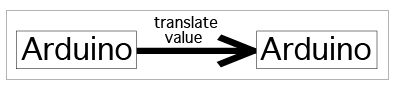## What Role Does the Sketch Play in Our Circuit?

You’ve seen the circuit in action, so you understand what it does, but how does the Arduino translate the potentiometer’s resistance value to a brightness value for the LED? To figure that out, let’s take a look at how electricity and information flow through our circuit.

#### Step 1: power into the Arduino5 volts of power come into the the Arduino from the computer via the USB cable.

#### Step 2: power into the potentiometer5 volts get sent to the one side of the potentiometer from the 5v pin on the Arduino (via the power bus).

#### Step 3: potentiometer changes the voltageThe potentiometer creates resistance, lowering the voltage, and then sends this new voltage back to the Arduino via pin A0.

#### Step 4: Arduino reads the voltageOn pin A0, the Arduino reads the voltage coming in from the potentiometer, and translates the voltage value to a number on the 0-1023 analog scale we mentioned earlier. Sometimes this reading takes a longer amount of time. We will talk about how the Arduino determines the value later on in the chapter.

#### Step 5: Arduino converts the valueThe Arduino changes the analog value from the potentiometer to a translated analog value using a function named map(), which we will explain later in the chapter. This step is crucial since the LED won’t understand values between 0 and 1023, but will accept values between 0 and 255.

#### Step 6: Arduino writes the value to the LEDThe Arduino sends this translated analog value to the LED through pin 9 using PWM. We will explain what PWM is and how it works later in the chapter.

#### Step 7: LED lights upThe LED lights up; how bright or dim it is will be determined by the analog value it received.

As you can see, the sketch performs the important step of translating the information from the potentiometer into a value that is used to control the brightness of the LED.

Now that we’ve seen an overview of what’s going on in the circuit and how it interacts with the sketch it’s time to dive into the details of the sketch.